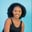Related Tags

pandas
python3

# How to use the Series.le() function in pandasMaria Elijah

## The Series.le() function

The pandas Series.le() function compares two series. This is done element-wise. It returns True if the Series a is less than or equal to Series b. Otherwise, it returns False.

### Syntax

Series.le(other, level=None, fill_value=None, axis=0)


### Parameters

• other: It represents the other Series to be compared.

• level (optional): The default is None. It represents the level (in the case of multilevel) to count along.

• fill_value: It represents the value to replace the missing values in the Series. If the element in both corresponding Series indexes is NaN, the result of filling at that specific index will be None. The default value is None.

• axis: This represents the axis to perform the operation on. Value 0 indicates rows and 1 indicates columns.

### Example

The following code demonstrates how to use the Series.le() function in pandas:

import pandas as pd
import numpy as np

# create Series
a = pd.Series([7, 12, 9, np.nan, 23, 7, np.nan])

b = pd.Series([10, np.nan, 28, 45, 73, 40, np.nan])

# value to fill nan
replace_nan = 5

# finds the smaller or equal to element between the two Series using Series.le()
print(a.le(b, fill_value=replace_nan))

### Explanation

• Line 1–2: We import the libraries pandas and numpy.

• Lines 5–7: We create two Series, a and b.

• Line 10: We create a variable replace_nan and assign a value to it. This replaces the NaN values in the Series.

• Line 13: We find smaller or equal elements between the Series a and b using the le() function.

Note: The default value for fill_value will be used if the element in both corresponding Series indexes is NaN.

RELATED TAGS

pandas
python3

CONTRIBUTORMaria Elijah
RELATED COURSES

View all Courses

Keep Exploring

Learn in-demand tech skills in half the time